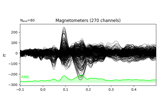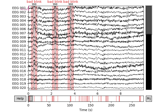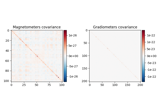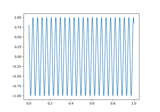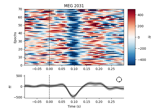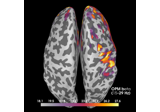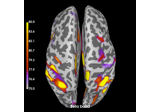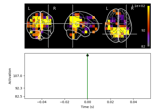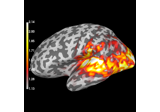# mne.compute_raw_covariance¶

mne.compute_raw_covariance(raw, tmin=0, tmax=None, tstep=0.2, reject=None, flat=None, picks=None, method='empirical', method_params=None, cv=3, scalings=None, n_jobs=1, return_estimators=False, reject_by_annotation=True, rank=None, verbose=None)[source]

Estimate noise covariance matrix from a continuous segment of raw data.

It is typically useful to estimate a noise covariance from empty room data or time intervals before starting the stimulation.

Note

To estimate the noise covariance from epoched data, use `mne.compute_covariance()` instead.

Parameters
rawinstance of `Raw`

Raw data.

tmin`float`

Beginning of time interval in seconds. Defaults to 0.

tmax

End of time interval in seconds. If None (default), use the end of the recording.

tstep`float` (default 0.2)

Length of data chunks for artifact rejection in seconds. Can also be None to use a single epoch of (tmax - tmin) duration. This can use a lot of memory for large `Raw` instances.

reject

Rejection parameters based on peak-to-peak amplitude. Valid keys are ‘grad’ | ‘mag’ | ‘eeg’ | ‘eog’ | ‘ecg’. If reject is None then no rejection is done. Example:

```reject = dict(grad=4000e-13, # T / m (gradiometers)
mag=4e-12, # T (magnetometers)
eeg=40e-6, # V (EEG channels)
eog=250e-6 # V (EOG channels)
)
```
flat

Rejection parameters based on flatness of signal. Valid keys are ‘grad’ | ‘mag’ | ‘eeg’ | ‘eog’ | ‘ecg’, and values are floats that set the minimum acceptable peak-to-peak amplitude. If flat is None then no rejection is done.

picks

Channels to include. Slices and lists of integers will be interpreted as channel indices. In lists, channel type strings (e.g., `['meg', 'eeg']`) will pick channels of those types, channel name strings (e.g., `['MEG0111', 'MEG2623']` will pick the given channels. Can also be the string values “all” to pick all channels, or “data” to pick data channels. None (default) will pick good data channels (excluding reference MEG channels). Note that channels in `info['bads']` will be included if their names or indices are explicitly provided.

method`str` | `list` | `None` (default ‘empirical’)

The method used for covariance estimation. See `mne.compute_covariance()`.

New in version 0.12.

method_params

Additional parameters to the estimation procedure. See `mne.compute_covariance()`.

New in version 0.12.

cv`int` | `sklearn.model_selection` object (default 3)

The cross validation method. Defaults to 3, which will internally trigger by default `sklearn.model_selection.KFold` with 3 splits.

New in version 0.12.

scalings

Defaults to `dict(mag=1e15, grad=1e13, eeg=1e6)`. These defaults will scale magnetometers and gradiometers at the same unit.

New in version 0.12.

n_jobs`int`

The number of jobs to run in parallel (default 1). Requires the joblib package.

New in version 0.12.

return_estimatorsbool (default `False`)

Whether to return all estimators or the best. Only considered if method equals ‘auto’ or is a list of str. Defaults to False.

New in version 0.12.

reject_by_annotationbool

Whether to reject based on annotations. If `True` (default), epochs overlapping with segments whose description begins with `'bad'` are rejected. If `False`, no rejection based on annotations is performed.

New in version 0.14.

rank`None` | ‘info’ | ‘full’ | `dict`

This controls the rank computation that can be read from the measurement info or estimated from the data. When a noise covariance is used for whitening, this should reflect the rank of that covariance, otherwise amplification of noise components can occur in whitening (e.g., often during source localization).

`None`

The rank will be estimated from the data after proper scaling of different channel types.

`'info'`

The rank is inferred from `info`. If data have been processed with Maxwell filtering, the Maxwell filtering header is used. Otherwise, the channel counts themselves are used. In both cases, the number of projectors is subtracted from the (effective) number of channels in the data. For example, if Maxwell filtering reduces the rank to 68, with two projectors the returned value will be 66.

`'full'`

The rank is assumed to be full, i.e. equal to the number of good channels. If a `Covariance` is passed, this can make sense if it has been (possibly improperly) regularized without taking into account the true data rank.

`dict`

Calculate the rank only for a subset of channel types, and explicitly specify the rank for the remaining channel types. This can be extremely useful if you already know the rank of (part of) your data, for instance in case you have calculated it earlier.

This parameter must be a dictionary whose keys correspond to channel types in the data (e.g. `'meg'`, `'mag'`, `'grad'`, `'eeg'`), and whose values are integers representing the respective ranks. For example, `{'mag': 90, 'eeg': 45}` will assume a rank of `90` and `45` for magnetometer data and EEG data, respectively.

The ranks for all channel types present in the data, but not specified in the dictionary will be estimated empirically. That is, if you passed a dataset containing magnetometer, gradiometer, and EEG data together with the dictionary from the previous example, only the gradiometer rank would be determined, while the specified magnetometer and EEG ranks would be taken for granted.

The default is `None`.

New in version 0.17.

New in version 0.18: Support for ‘info’ mode.

verbose

If not None, override default verbose level (see `mne.verbose()` and Logging documentation for more). If used, it should be passed as a keyword-argument only.

Returns
covinstance of `Covariance` | `list`

The computed covariance. If method equals ‘auto’ or is a list of str and return_estimators equals True, a list of covariance estimators is returned (sorted by log-likelihood, from high to low, i.e. from best to worst).

`compute_covariance`

Estimate noise covariance matrix from epoched data.

Notes

This function will:

1. Partition the data into evenly spaced, equal-length epochs.

2. Load them into memory.

3. Subtract the mean across all time points and epochs for each channel.

4. Process the `Epochs` by `compute_covariance()`.

This will produce a slightly different result compared to using `make_fixed_length_events()`, `Epochs`, and `compute_covariance()` directly, since that would (with the recommended baseline correction) subtract the mean across time for each epoch (instead of across epochs) for each channel.

## Examples using `mne.compute_raw_covariance`¶